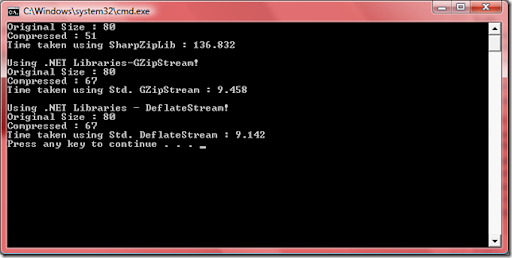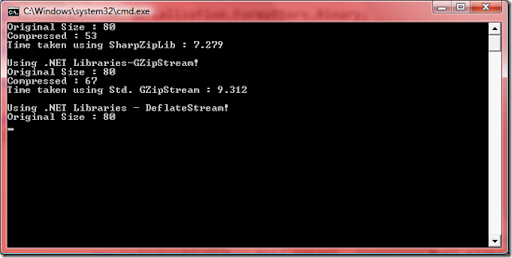## Monday, March 16, 2009

### Compression libraries in C# : Some observations

I wanted to see how long it takes for large data to be compressed in memory (thought it would be efficient). So I tried to use ICSharpCode SharpZipLib and also compared the efficiency with GZipStream/DeflateStream. I used my TimeAndExecute() method I posted earlier and used a 80 MB random data.

My observations are:

1. There is no CompressionLevel setting to be used with GZipStream/DeflateStream.

2. CompressionLevel when set in SharpZipLib has good impact. BEST_SPEED was way 2 seconds faster than the other two standard BCL classes.

3. DeflateStream is faster than GZipStream.

Code used:

`   1: public class ZipHelper`

`   2:     {`

`   3:         private static readonly int BUFF_SIZE = 1024 * 1024 * 1;`

`   4:  `

`   5:         public static byte[] Compress(byte[] data)`

`   6:         {`

`   7:             var memory = new MemoryStream();`

`   8:             using (DeflaterOutputStream stream = new DeflaterOutputStream(memory,`

`   9:                 new Deflater(Deflater.BEST_COMPRESSION), ZipHelper.BUFF_SIZE))`

`  10:             {`

`  11:                 stream.Write(data, 0, data.Length);`

`  12:             }`

`  13:             return memory.ToArray();`

`  14:         }`

`  15:  `

`  16:         public static byte[] StdCompress(byte[] data)`

`  17:         {`

`  18:             var memory = new MemoryStream();`

`  19:             using (var strm = new GZipStream(memory, CompressionMode.Compress))`

`  20:             {`

`  21:                 strm.Write(data, 0, data.Length);`

`  22:             }`

`  23:             return memory.ToArray();`

`  24:         }`

`  25:  `

`  26:         public static byte[] DeflateCompress(byte[] data)`

`  27:         {`

`  28:             var memory = new MemoryStream();`

`  29:             using (var strm = new DeflateStream(memory, CompressionMode.Compress))`

`  30:             {`

`  31:                 strm.Write(data, 0, data.Length);`

`  32:             }`

`  33:             return memory.ToArray();`

`  34:         }`

`  35:  `

`  36:         public static byte[] GetBytes(object o)`

`  37:         {`

`  38:             using (MemoryStream mem = new MemoryStream(ZipHelper.BUFF_SIZE))`

`  39:             {`

`  40:                 var bf = new BinaryFormatter();`

`  41:                 bf.Serialize(mem, o);`

`  42:                 return mem.ToArray();`

`  43:             }`

`  44:         }`

`  45:  `

`  46:         public static byte[] DeflateCompress(object obj)`

`  47:         {`

`  48:             return DeflateCompress(GetBytes(obj));`

`  49:         }`

`  50:         public static byte[] StdCompress(object obj)`

`  51:         {`

`  52:             return StdCompress(GetBytes(obj));`

`  53:         }`

`  54:  `

`  55:         public static byte[] Compress(object o)`

`  56:         {`

`  57:             return Compress(GetBytes(o));`

`  58:         }`

`  59:  `

`  60:     }`

Using SharpZipLib CompressionMode.BEST_COMPRESSIONUsing SharpZipLib CompressionMode.BEST_SPEEDSo clearly, it is not a good idea to use compressed data that is frequently accessed in memory. It takes more time to compress as well as decompress. I did not bother with de-compression though! Extensions later.